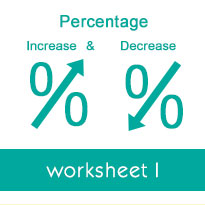# Comparing discounts: Worksheets

Welcome to the Percentage Increase and Decrease Worksheets section at Tutorialspoint.com. On this page, you will find worksheets on finding the final amount given the original amount and a percentage increase or decrease, finding the sale price given the original price and percent discount, finding the sale price without a calculator given the original price and percent discount, finding the total cost including tax or markup, finding the original amount given the result of a percentage increase or decrease, finding the original price given the sale price and percent discount, comparing discounts and so on.

To practice Math skills, there is nothing more effective than solving worksheets. Our free to download, printable worksheets help you practice Math concepts, and improve your analytical and problem-solving skills. We have 1 worksheets for each topic in the tutorial.